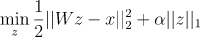# Grad not updating, (solving MSE with L1 regularization, useing torch as GD)

im trying to find the argmin of this equation:And because there is no analytical solution for this problem, im trying to use torch as my GD. and find the minimum.

where (W) is the toeplitz matrix of the original audio (with no noise)
(x) is my attempt to cleaning the audio from an acoustic noise (x = h_original * audio_original)
and what i want ot find is (z), when (z) is the room impulse response im trying to find, and then comper it to the real h

(in this code, W = Toeplitz_x, z = h_audio, x = y_cpu)

so i have build a small pytorch code inorder to find this h

``````            h_audio = torch.randn(len(audio)).reshape(-1, 1)
lr = 0.1
lossMSE = nn.MSELoss()
stop = 1

forward = torch.matmul(Toeplitz_x, h_audio)
y_cpu = audio.to('cpu').reshape(-1,1)

loss = lossMSE(forward, y_cpu) + LAMBDA * torch.sum(torch.abs(h_audio))
while stop < loss:
loss.backward(retain_graph=True)
optimizer.step()